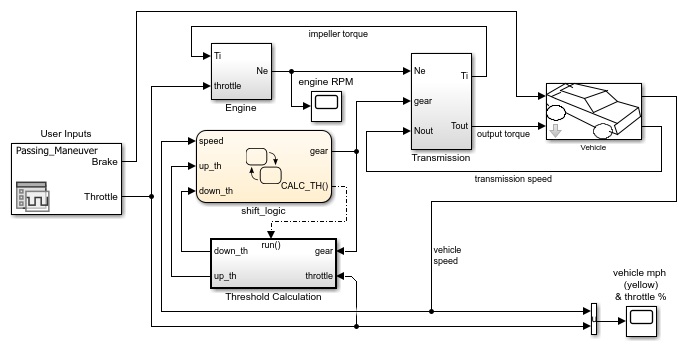## Design Charts with Simulink Functions

In this tutorial, you use a Simulink® function in a Stateflow® chart to improve the design of a model that contains a function-call subsystem. You can replace a function-call subsystem with a Simulink function in a chart when:

• The subsystem performs calculations required by the chart.

• Other blocks in the model do not need access to the subsystem outputs.

Simulink functions are not supported in standalone Stateflow charts in MATLAB®.

Note

To skip the conversion steps, open the model `sf_car`:

`openExample("stateflow/AutomaticTransmissionWithActiveStateDataExample")`

### Open the Model

Open the model `old_sf_car`. This model contains a function-call subsystem named `Threshold Calculation` and a Stateflow chart named `shift_logic`.When you run this model, the chart broadcasts the output event `CALC_TH` to trigger the function-call subsystem. The subsystem interpolates two values for the `shift_logic` chart. The subsystem outputs (`up_th` and `down_th`) return to the chart as inputs.

After the simulation, the Scope blocks display these results.1. In the Simulink model, right-click the Threshold Calculation block in the lower left corner and select Cut from the context menu.2. Open the shift_logic chart.

3. In the chart, right-click below `selection_state` and select Paste from the context menu.

4. Expand the new Simulink function so that the signature fits inside the function box.Tip

To change the font size of a function, right-click the function box and select a new size from the Font Size menu.

5. Expand the border of `selection_state` to include the new function.Note

The function resides in this state instead of the chart level because no other state in the chart requires the function outputs `up_th` and `down_th`. See Bind a Simulink Function to a State.

6. Rename the Simulink function from `Threshold_Calculation` to `calc_threshold` by entering ```[down_th, up_th] = calc_threshold(gear, throttle)``` in the function box.

### Change the Scope of Chart Data

In the Model Explorer, change the scope of chart-level data `up_th` and `down_th` to `Local` because calculations for those data now occur inside the chart.

### Update State Action in the Chart

In the Stateflow Editor, change the `during` action in `selection_state` to call the Simulink function `calc_threshold`.

`during: [down_th, up_th] = calc_threshold(gear, throttle);`### Add Data to the Chart

Because the function `calc_threshold` takes `throttle` as an input, you must define that data as a chart input. (For details, see Add Stateflow Data.)

1. Add input data `throttle` to the chart with a Port property of 1.

Using port 1 prevents signal lines from overlapping in the Simulink model.

2. In the Simulink model, add a signal line for `throttle` to an input to the Engine block and to an input to the shift_logic chart.

### Remove Unused Items in the Model

1. In the Model Explorer, delete the function-call output event `CALC_TH` because the Threshold Calculation block no longer exists.

2. Delete any dashed signal lines from your model.

### Run the New Model

Your new model looks something like this:If you simulate the new model, the results match those of the original design.